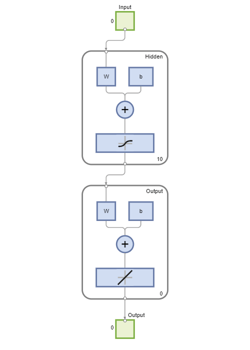# fitnet

Function fitting neural network

## Syntax

``net = fitnet(hiddenSizes)``
``net = fitnet(hiddenSizes,trainFcn)``

## Description

example

````net = fitnet(hiddenSizes)` returns a function fitting neural network with a hidden layer size of `hiddenSizes`.```

example

````net = fitnet(hiddenSizes,trainFcn)` returns a function fitting neural network with a hidden layer size of `hiddenSizes` and training function, specified by `trainFcn`.```

## Examples

collapse all

```[x,t] = simplefit_dataset; ```

The 1-by-94 matrix `x` contains the input values and the 1-by-94 matrix `t` contains the associated target output values.

Construct a function fitting neural network with one hidden layer of size 10.

```net = fitnet(10); ```

View the network.

```view(net) ```The sizes of the input and output are zero. The software adjusts the sizes of these during training according to the training data.

Train the network `net` using the training data.

```net = train(net,x,t); ```

View the trained network.

```view(net) ```You can see that the sizes of the input and output are 1.

Estimate the targets using the trained network.

```y = net(x); ```

Assess the performance of the trained network. The default performance function is mean squared error.

```perf = perform(net,y,t) ```
```perf = 1.4639e-04 ```

The default training algorithm for a function fitting network is Levenberg-Marquardt ( `'trainlm'` ). Use the Bayesian regularization training algorithm and compare the performance results.

```net = fitnet(10,'trainbr'); net = train(net,x,t); y = net(x); perf = perform(net,y,t) ```
```perf = 3.3261e-10 ```

The Bayesian regularization training algorithm improves the performance of the network in terms of estimating the target values.

## Input Arguments

collapse all

Size of the hidden layers in the network, specified as a row vector. The length of the vector determines the number of hidden layers in the network.

Example: For example, you can specify a network with 3 hidden layers, where the first hidden layer size is 10, the second is 8, and the third is 5 as follows: `[10,8,5]`

The input and output sizes are set to zero. The software adjusts the sizes of these during training according to the training data.

Data Types: `single` | `double`

Training function name, specified as one of the following.

Training FunctionAlgorithm
`'trainlm'`

Levenberg-Marquardt

`'trainbr'`

Bayesian Regularization

`'trainbfg'`

BFGS Quasi-Newton

`'trainrp'`

Resilient Backpropagation

`'trainscg'`

`'traincgb'`

`'traincgf'`

`'traincgp'`

`'trainoss'`

One Step Secant

`'traingdx'`

`'traingdm'`

`'traingd'`

Example: For example, you can specify the variable learning rate gradient descent algorithm as the training algorithm as follows: `'traingdx'`

For more information on the training functions, see Train and Apply Multilayer Shallow Neural Networks and Choose a Multilayer Neural Network Training Function.

Data Types: `char`

## Output Arguments

collapse all

Function fitting network, returned as a `network` object.

## Tips

• Function fitting is the process of training a neural network on a set of inputs in order to produce an associated set of target outputs. After you construct the network with the desired hidden layers and the training algorithm, you must train it using a set of training data. Once the neural network has fit the data, it forms a generalization of the input-output relationship. You can then use the trained network to generate outputs for inputs it was not trained on.